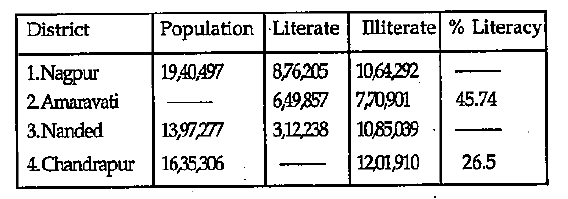# Data Interpretation - Tabular Data

>>>>>>>>Tabular Data

Instructions:

Refer the following table and answer questions below• A

45.27%• B

45.26%• C

22.35%• D

26.77%Instructions:

Refer the following table and answer questions below• A

1984• B

1985• C

1986• D

1987• Option : C
• Explanation : For E type car, the ratio of rejection to production in 1983 = 0.067

Similar figure for other ahead years are 0.069; 0.056, 0.038, 0.044, 0.050 respectively

Instructions:

Refer the following table and answer questions below• A

Less• B

More• C

The same• D

None of the aboveInstructions:

Refer the following table and answer questions below• A

1,27,65,242• B

41,22,142• C

1,16,65,242• D

1,66,15,524Instructions:

Refer the following table and answer questions below• A

Nagpur district• B

Amaravati district• C

Nanded district• D

None of theseRelated Quiz.
Tabular Data